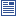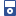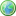Accessibility options:

# Arithmetic resources

Show me all resources applicable to

### Community Project (1)Mathematical Symbols and Abbrevations SOURCE
A zip file containing LaTeX source and eps files for the quick reference leaflet 'Mathematical Symbols and Abbrevations' contributed to the mathcentre Community Project by Janette Matthews and reviewed by Tony Croft, Loughborough University.

### Facts & Formulae Leaflets (2)Facts & Formulae for Functional Mathematics - Onscreen version
A onscreen version of the Fact & Formulae leaflet for Functional Mathematics contributed to the mathcentre Community Project by Anne Townsend.Facts & Formulae for Functional Mathematics - Print version
A Fact & Formulae leaflet for Functional Mathematics contributed to the mathcentre Community Project by Anne Townsend. This leaflet is designed to be printed landscape on A4 and folded.

### iPOD Video (15)Adding and Subtracting Fractions Part 1
This video segment introduces the addition and subtraction of fractions. This resource is released under a Creative Commons license Attribution-Non-Commercial-No Derivative Works and the copyright is held by Skillbank Solutions Ltd.Adding and Subtracting Fractions Part 5
This video segment shows the addition of three mixed fractions. This resource is released under a Creative Commons license Attribution-Non-Commercial-No Derivative Works and the copyright is held by Skillbank Solutions Ltd.Fractions - basic ideas Part 1 - what is a fraction ?
This iPod download is part of a video tutorial on Fractions. It explains what is meant by a fraction, and gives some examples. It shows equivalent fractions - that is fractions having the same value, but written in different forms. This resource is released under a Creative Commons license Attribution-Non-Commercial-No Derivative Works and the copyright is held by Skillbank Solutions Ltd.Fractions - Multipying and Dividing - Part 1
This video segment introduces the multiplication and division of fractions. This resource is released under a Creative Commons license Attribution-Non-Commercial-No Derivative Works and the copyright is held by Skillbank Solutions Ltd.Fractions - Multipying and Dividing - Part 2
This video further illustrates the multiplication and division of fractions. This resource is released under a Creative Commons license Attribution-Non-Commercial-No Derivative Works and the copyright is held by Skillbank Solutions Ltd.Fractions - Multipying and Dividing - Part 3
This video further illustrates the multiplication and division of fractions. This resource is released under a Creative Commons license Attribution-Non-Commercial-No Derivative Works and the copyright is held by Skillbank Solutions Ltd.Fractions - Multipying and Dividing - Part 4
This video further illustrates the multiplication and division of fractions. This resource is released under a Creative Commons license Attribution-Non-Commercial-No Derivative Works and the copyright is held by Skillbank Solutions Ltd.Fractions - Multipying and Dividing - Part 5
This video further illustrates the multiplication and division of fractions. This resource is released under a Creative Commons license Attribution-Non-Commercial-No Derivative Works and the copyright is held by Skillbank Solutions Ltd.Fractions - Multipying and Dividing - Part 6
This video further illustrates the multiplication and division of fractions. This resource is released under a Creative Commons license Attribution-Non-Commercial-No Derivative Works and the copyright is held by Skillbank Solutions Ltd.Fractions - Multipying and Dividing - Part 7
This video further illustrates the multiplication and division of fractions. This resource is released under a Creative Commons license Attribution-Non-Commercial-No Derivative Works and the copyright is held by Skillbank Solutions Ltd.Fractions - Multipying and Dividing - Part 8
This video further illustrates the multiplication and division of fractions. This resource is released under a Creative Commons license Attribution-Non-Commercial-No Derivative Works and the copyright is held by Skillbank Solutions Ltd.Percentages - part 1
This video segment introduces percentages. This resource is released under a Creative Commons license Attribution-Non-Commercial-No Derivative Works and the copyright is held by Skillbank Solutions Ltd.Percentages - part 2
This video segment develops the material in the previous unit on percentages. This resource is released under a Creative Commons license Attribution-Non-Commercial-No Derivative Works and the copyright is held by Skillbank Solutions Ltd.Percentages - part 3
This unit develops the previous unit on percentages. This resource is released under a Creative Commons license Attribution-Non-Commercial-No Derivative Works and the copyright is held by Skillbank Solutions Ltd.Percentages - part 4
This unit develops the previous unit on percentages. This resource is released under a Creative Commons license Attribution-Non-Commercial-No Derivative Works and the copyright is held by Skillbank Solutions Ltd.

### Motivating Mathematics (1)minus x minus = plus - Mike Savage
This mathtutor extention video explains how multiplying a negative number by another negative number gives a positive number. This resource is released under a Creative Commons license Attribution-Non-Commercial-No Derivative Works and the copyright is held by Skillbank Solutions Ltd.

### Practice & Revision (1)Algebra Refresher - Interactive version
An interactive version of the refresher booklet on Algebra including links to other resources for further explanation. It includes revision, exercises and solutions on fractions, indices, removing brackets, factorisation, algebraic frations, surds, transpostion of formulae, solving quadratic equations and some polynomial equations, and partial fractions. An interactive version and a welsh language version are available.

### Quick Reference (8)Addition and subtraction
Fractions involving symbols occur frequently. It is necessary to be able to add and subtract them. On this leaflet, we revise how these processes are carried out. An understanding of writing fractions in equivalent forms is necessary.Decimals
This leaflet shows how easy it is to multiply or divide decimals by powers of ten.Fractions
The ability to work confidently with fractions, both number fractions and algebraic fractions, is an essential skill which underpins all other algebraic processes. In this leaflet, we remind you how number fractions are simplified, added, subtracted, multiplied and divided.Mathematical Symbols and Abbreviations
This leaflet provides information on symbols and notation commonly used in mathematics. It shows the meaning of a symbol and, where necessary, an example and an indication of how the symbol would be said. For further information from mathcentre resources, a search phrase is given. This Quick Reference leaflet is contributed to the mathcentre Community Project by Janette Matthews and reviewed by Tony Croft, University of Loughborough.Multiplication And Division
Fractions involving symbols occur frequently. It is necessary to be able to multiply and divide them. On this leaflet we revise how these processes are carried out.Percentages
The use of percentages is common in many aspects of commercial life. Interest rates, discounts, pay rises and so on, are all expressed using percentages. This leaflet revises the meaning of the term 'percentage', and shows how to calculate percentages, and how to convert expressions involving percentages into alternative forms.Ratios
Ratios are an alternative way of expressing fractions. This leaflet will revise ratio calculationsSigma notation
Sigma notation provides a concise and convenient way of writing long sums. This leaflet explains how.

### Teach Yourself (4)Decimals
In this unit we shall look at the meaning of decimals, and how they are related to fractions. We shall then look at rounding to given numbers of decimal places or significant figures. Finally we shall take a brief look at irrational numbers.Percentages
In this unit we shall look at the meaning of percentages and carry out calculations involving percentages. We will also look at the use of the percentage button on calculators.Ratios
A ratio is a way of comparing two or more similar quantities, by writing two or more numbers separated by colons. The numbers should be whole numbers, and should not include units.Sigma notation
Sigma notation is a method used to write out a long sum in a concise way. In this unit we look at ways of using sigma notation, and establish some useful rules.

### Test Yourself (1)Ratios Test 01 (DEWIS)
Three questions involving ratios. DEWIS resources have been made available under a Creative Commons licence by Rhys Gwynllyw & Karen Henderson, University of the West of England, Bristol.

### Video (5)Fractions - adding and subtracting
In this unit we shall see how to add and subtract fractions. We shall also see how to add and subtract mixed fractions by turning them into improper fractions. (Mathtutor Video Tutorial) This resource is released under a Creative Commons license Attribution-Non-Commercial-No Derivative Works and the copyright is held by Skillbank Solutions Ltd.Fractions - basic ideas
What are fractions? Fractions are ways of writing parts of whole numbers. For example if we take a pizza, and divide it up equally between 4 people, each person will have 1/4 or, written in words, one quarter of the pizza. (Mathtutor Video Tutorial) This resource is released under a Creative Commons license Attribution-Non-Commercial-No Derivative Works and the copyright is held by Skillbank Solutions Ltd.Fractions - multiplying and dividing
In this unit we shall see how to multiply fractions. We shall also see how to divide fractions by turning the second fraction upside down. (Mathtutor Video Tutorial) This resource is released under a Creative Commons license Attribution-Non-Commercial-No Derivative Works and the copyright is held by Skillbank Solutions Ltd.Mathematical language
This introductory section provides useful background material on the importance of symbols in mathematical work. It describes conventions used by mathematicians, engineers, and scientists. (Mathtutor Video Tutorial) This resource is released under a Creative Commons license Attribution-Non-Commercial-No Derivative Works and the copyright is held by Skillbank Solutions Ltd.Percentages
In this unit we shall look at the meaning of percentages and carry out calculations involving percentages. We will also look at the use of the percentage button on calculators. (Mathtutor Video Tutorial) This resource is released under a Creative Commons license Attribution-Non-Commercial-No Derivative Works and the copyright is held by Skillbank Solutions Ltd.

### Video with captions which require edits (7)Arithmetic and Geometric Progressions
This unit introduces sequences and series, and gives some simple examples of each. It also explores particular types of sequence known as arithmetic progressions (APs) and geometric progressions (GPs), and the corresponding series. (Mathtutor Video Tutorial) The video is released under a Creative Commons license Attribution-Non-Commercial-No Derivative Works and the copyright is held by Skillbank Solutions Ltd.Fractions - adding and subtracting
In this unit we shall see how to add and subtract fractions. We shall also see how to add and subtract mixed fractions by turning them into improper fractions. (Mathtutor Video Tutorial) This resource is released under a Creative Commons license Attribution-Non-Commercial-No Derivative Works and the copyright is held by Skillbank Solutions Ltd.Fractions - basic ideas
What are fractions? Fractions are ways of writing parts of whole numbers. For example if we take a pizza, and divide it up equally between 4 people, each person will have 1/4 or, written in words, one quarter of the pizza. (Mathtutor Video Tutorial) This resource is released under a Creative Commons license Attribution-Non-Commercial-No Derivative Works and the copyright is held by Skillbank Solutions Ltd.Fractions - multiplying and dividing
In this unit we shall see how to multiply fractions. We shall also see how to divide fractions by turning the second fraction upside down. (Mathtutor Video Tutorial) This resource is released under a Creative Commons license Attribution-Non-Commercial-No Derivative Works and the copyright is held by Skillbank Solutions Ltd.Mathematical language
This introductory section provides useful background material on the importance of symbols in mathematical work. It describes conventions used by mathematicians, engineers, and scientists. (Mathtutor Video Tutorial) The video is released under a Creative Commons license Attribution-Non-Commercial-No Derivative Works and the copyright is held by Skillbank Solutions Ltd.minus x minus = plus - Mike Savage
This mathtutor extention video explains how multiplying a negative number by another negative number gives a positive number. The video is released under a Creative Commons license Attribution-Non-Commercial-No Derivative Works and the copyright is held by Skillbank Solutions Ltd.Percentages
In this unit we shall look at the meaning of percentages and carry out calculations involving percentages. We will also look at the use of the percentage button on calculators. (Mathtutor Video Tutorial) The video is released under a Creative Commons license Attribution-Non-Commercial-No Derivative Works and the copyright is held by Skillbank Solutions Ltd.